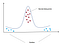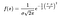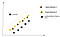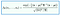# Day 27 — Handling of Outliers using Gaussian Distribution(probability density function)

Till now we have seen anomaly detection as unsupervised learning where we do not have any target data to identify whether an input sample is an outlier. On the other hand, when we have non-outlier(desired) data at hand, then we can leverage the supervised learning to detect the anomalies. For instance, Credit Card fraud detection to figure out any anomalous data. Since we are already aware of the non-oddity data patterns, it can be used to fit the model during the training. Any new patterns that are not introduced to the ML model already will be treated as outliers.

Table of contents:

1. Univariate — Probability density function
2. Multivariate — Gaussian distribution
3. Advantages & DisAdvantages

Univariate — Probability density function: Recollecting the normal distribution curve, where most of the data is occupied within 2 standard deviations (around 95%). If any data point falls outside this limit we categorize it as an abnormality. But this parameter can be set and usually termed as epsilon. It can be equated to the area under the curve(probability) for the distribution. If the computed probability density is less than the cut-off limit(epsilon), then the corresponding input data will be termed as deviation or irregularity.Fig1 — shows probability density function for outlier detection

We already know the probability density function of Gaussian distribution is,Pde for Gaussian from wiki

where sigma = standard deviation and mu = mean. During the training(learning), the value of mean and standard deviation is evaluated. These values act as a benchmark for future data. If the incoming new sample has a minimal value of probability, then the data point is an outlier. Whereas, if the data fits into the 95% (majority) of the curve, then there will not be any attention required.

Multivariate — Gaussian distribution: In practice, we receive multiple features(multivariate) as inputs to the anomaly detection algorithm. Multivariate Gaussian technique is an extension of the univariate method, involving more than one input features. The individual means corresponding to each independent variable is evaluated to apply on the formula. And instead of the standard deviation, we will be calculating the covariance matrix.

The intuition behind using the covariance matrix is, it captures the linear relation between the variables. If both the features are changing in the same direction then the covariance will be a maximum value. Contrastingly, if there is no link between the input features, then the covariance value will be zero.Fig 2 — shows the covariance effect w.r. feature 1 & 2

The probability density function can be altered to accommodate the multivariate case as below.The formula for the multivariate case from wiki

mu — represents the mean vector and sigma — the covariance matrix. |sigma| will be the determinant of the matrix.

Advantages:

1. When the input features have a linear relationship, then it helps to identify the outliers quickly without demanding high computation.
2. Easy interpretation and a straight forward technique.

Disadvantages:

1. Since the real-life data presents non-linear relationships, it might not work as expected for those scenarios.

Recommended Reading:

AI Enthusiast | Blogger✍

## More from Nandhini N

AI Enthusiast | Blogger✍